﻿ 二自由度水下云台自适应控制方法
 舰船科学技术2022, Vol. 44Issue (14): 89-94    DOI: 10.3404/j.issn.1672-7649.2022.14.020PDF

1. 天津科技大学 机械工程学院，天津 300222;
2. 河北工业大学 机械工程学院，天津 300131;
3. 天津瀚海蓝帆海洋科技有限公司，天津 300457

Research on adaptive control method of 2-DOF underwater pan-tilt
WANG Xiao-ming1, WANG Cheng-cai1, WU Tian-long2, WANG Chang-qiang3
1. School of Mechanical Engineering, Tianjin University of Science and Technology, Tianjin 300222, China;
2. School of Mechanical Engineering, Hebei University of Technology, Tianjin 300131, China;
3. Tianjin Hanhai Lanfan Marine Technology Co., Ltd., Tianjin 300457, China
Abstract: In order to solve the attitude stabilization problem of shipborne multi-beam sonar, a two-degree-of-freedom underwater pan-tilt is designed. On the basis of retaining the traditional underwater pan-tilt positioning mode, an adaptive mode was added to compensate the influence of wave on sonar attitude, and a step velocity PID adaptive control method was proposed. In this method, a matching velocity gradient is selected according to the angle of distance from the target, and then PID is introduced to adjust the underwater pan-tilt in real time, so that it can maintain a specific angle relative to the underwater pan-tilt through self-adjustment. According to the results of the underwater simulation experiment, both the two degrees of freedom of the underwater pan-tilt can reach the target angle at a speed of 18°/s, and the angle error is 0.4°. In the adaptive mode, the pitch degree of freedom with sonar can be stabilized at the hold angle in a short time, and the hold error is 1°, which proves the effectiveness of the control method.
Key words: Underwater pan-tilt     adaptive control     2-DOF     step speed     PID
0 引　言

1 水下云台物理系统 1.1 机械系统设计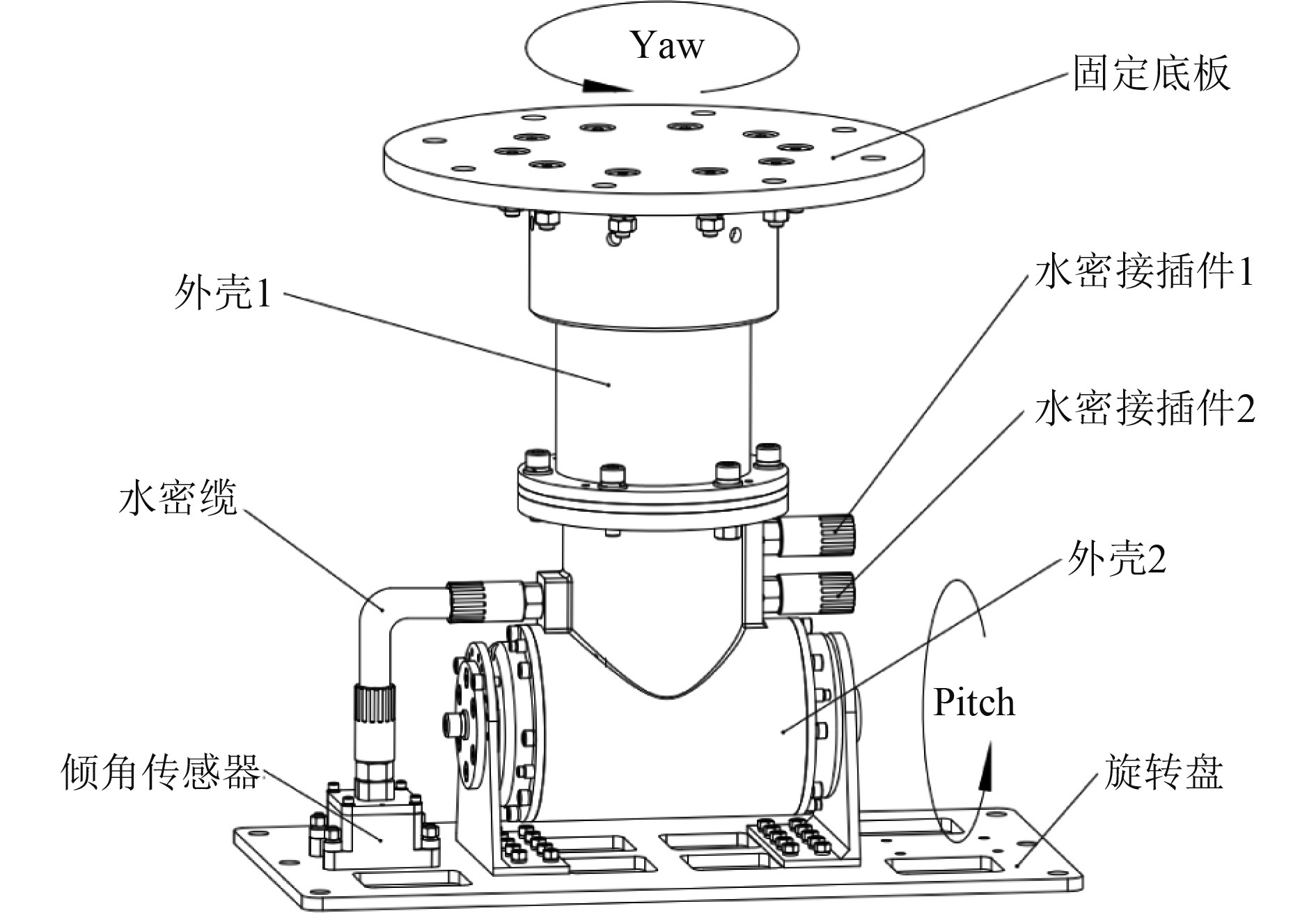图 1 云台机械结构图 Fig. 1 The mechanical structure drawing of pan-tilt
1.2 控制系统设计

1.2.1 硬件方案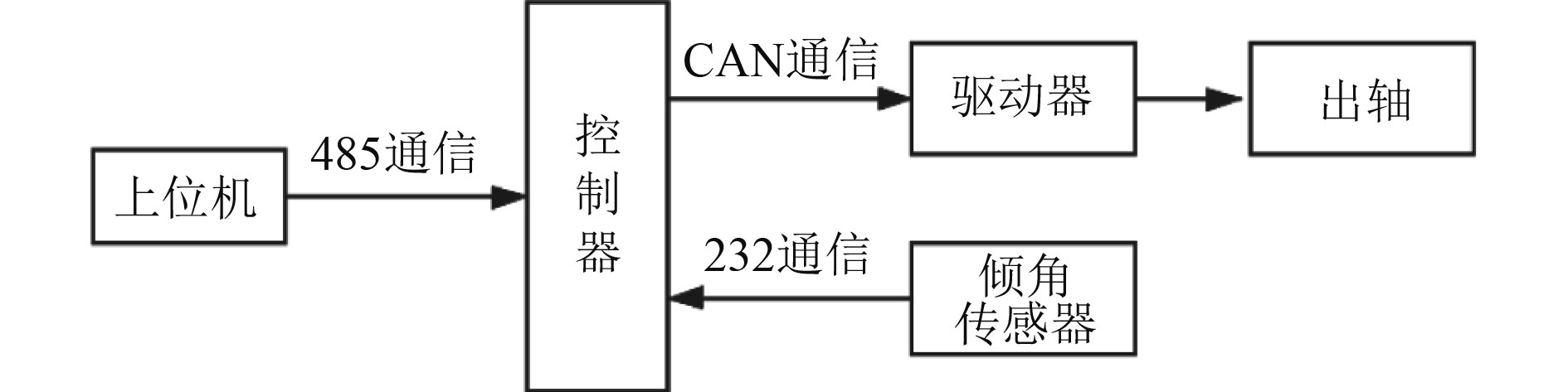图 2 云台硬件框图 Fig. 2 The hardware block diagram of pan-tilt

1.2.2 软件方案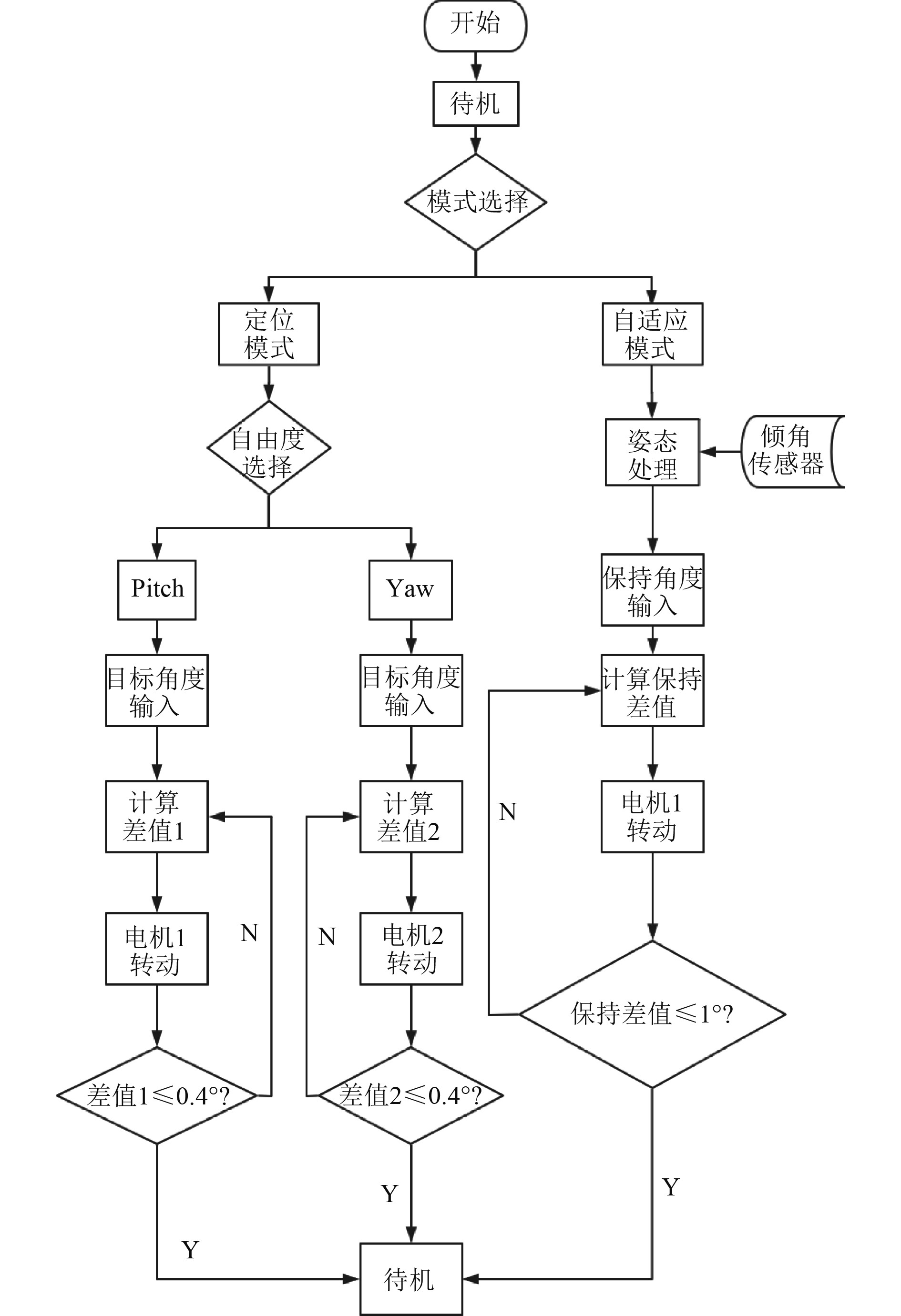图 3 云台控制程序框图 Fig. 3 The control program block diagram of pan-tilt

2 水下云台控制方法 2.1 定位模式

 $\Delta \left( t \right) = {\theta _m} - {\theta _n}\left( t \right)。$ (1)

 $\begin{array}{l} v\left(t\right)=\dfrac{\omega \left(t\right)}{i}=\dfrac{\Delta \left(t\right)}{i\times t}=\\ \Bigg\{\begin{array}{c}k\text{，}\\ mt+k\text{，}\\ 0\text{，}\end{array}\begin{array}{c}\\ \\ \end{array}\begin{array}{c}\left|\Delta \left(t\right)\right|\geqslant 10^{\circ}，\\ error < \left|\Delta \left(t\right)\right| < 10^{\circ}，\\ \left|\Delta \left(t\right)\right|\leqslant error。\end{array}\end{array}$ (2)

 $\left| k \right| = \frac{{\omega \left( t \right)}}{i} = \frac{{3\;600 \times f}}{{i \times p}} \approx 25.714f 。$ (3)

 $\begin{gathered} {\theta _n}\left( t \right) = \int {v\left( t \right)} {\rm{d}} t = \\ \left\{ \begin{gathered} kt,\begin{array}{*{20}{c}} {}&{}&{\begin{array}{*{20}{c}} {}&{\left| {\Delta \left( t \right)} \right| \geqslant 10^\circ } ，\end{array}} \end{array} \\ m{t^2} + kt + l,error < \left| {\Delta \left( t \right)} \right| < 10^\circ ，\\ {\theta _m},\begin{array}{*{20}{c}} {}&{}&{\begin{array}{*{20}{c}} {}&{\left| {\Delta \left( t \right)} \right| \leqslant error}。\end{array}} \end{array} \\ \end{gathered} \right. \\ \end{gathered}$ (4)

2.2 自适应模式

 ${\theta _m}\left( {t + T} \right) = {\theta _n}\left( t \right) + U\left( t \right) 。$ (5)

 \begin{aligned}[b] & U\left( t \right) = {K_p} \times e\left( t \right) + {K_i} \times \sum\limits_{n = 0}^t {e\left( n \right)} + \\ & {K_d} \times \left( {e\left( t \right) - e\left( {t - 1} \right)} \right) 。\end{aligned} (6)

 $e\left( t \right) = {\theta _c} - {\theta _q}\left( t \right) 。$ (7)

 $\begin{gathered} {\theta _m}\left( {t + T} \right) = {\theta _n}\left( t \right) + {K_i} \times \sum\limits_{n = 0}^t {e\left( n \right)} \\ \left\{ \begin{gathered} kt + {K_i} \times \sum\limits_{n = 0}^t {e\left( n \right),\begin{array}{*{20}{c}} {}&{}&{\begin{array}{*{20}{c}} {}&{\left| {e\left( t \right)} \right| \geqslant 10^\circ }，\end{array}} \end{array}} \\ m{t^2} + kt + l + {K_i} \times \sum\limits_{n = 0}^t {e\left( n \right),{\text{1}}^\circ < \left| {e\left( t \right)} \right| < 10^\circ } ，\\ {\theta _q},\begin{array}{*{20}{c}} {}&{}&{}&{\begin{array}{*{20}{c}} {}&{}&{\begin{array}{*{20}{c}} {}&{\begin{array}{*{20}{c}} {}&{\left| {e\left( t \right)} \right|\leqslant {\text{1}}^\circ } 。\end{array}} \end{array}} \end{array}} \end{array} \\ \end{gathered} \right. \\ \end{gathered}$ (8)

3 实　验

3.1 实验系统组成表 1 D5061参数表 Tab.1 The parameter table of D5061
3.2 定位模式实验

1）Yaw自由度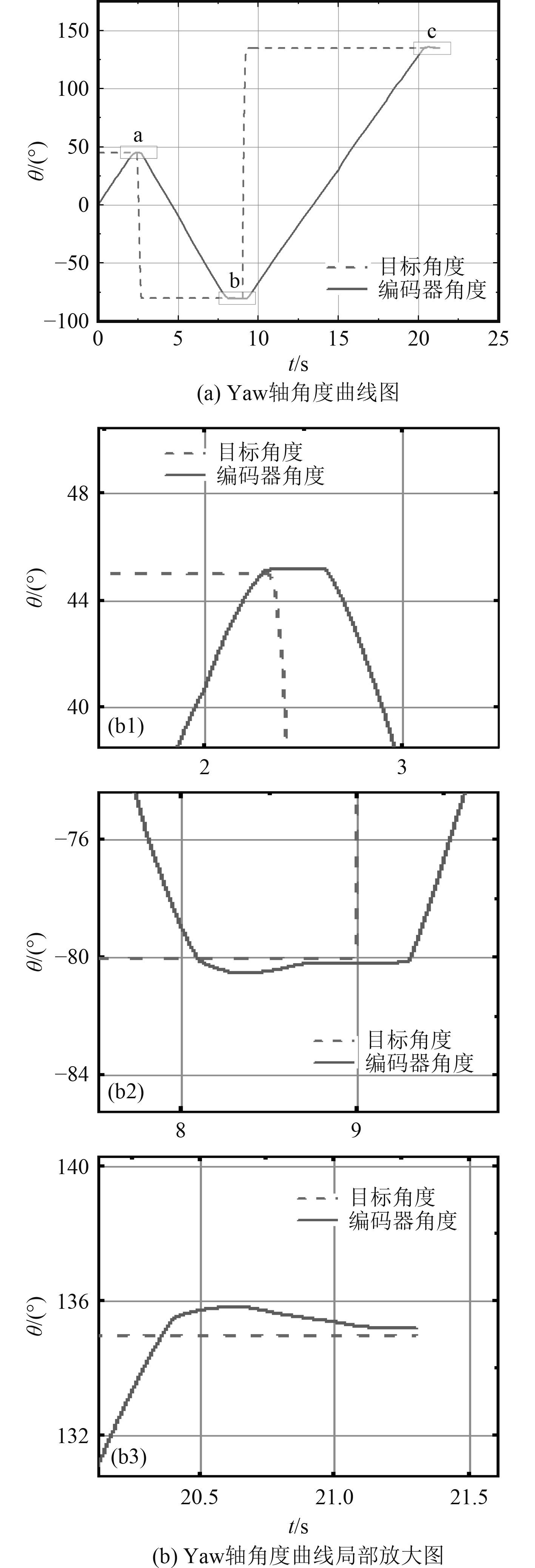图 4 定位模式Yaw轴目标角度与编码器角度 Fig. 4 Yaw axis target angle and encoder angle of positioning mode

2）Pitch自由度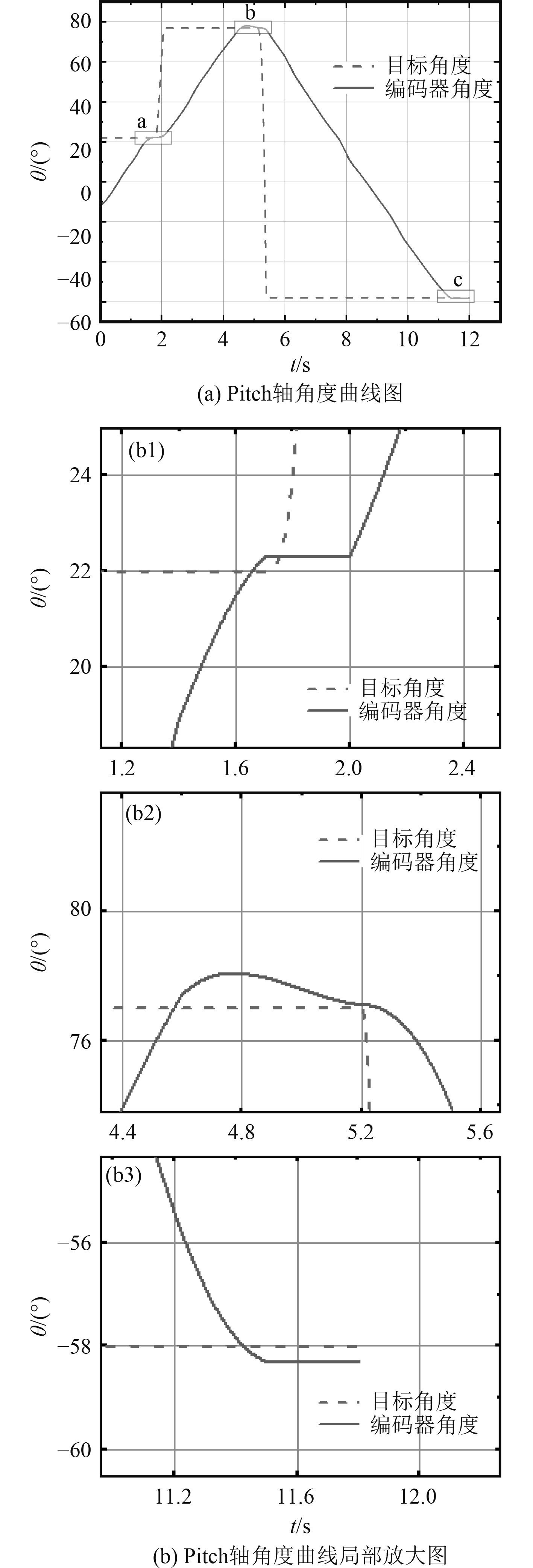图 5 定位模式Pirch轴目标角度与编码器角度 Fig. 5 Pitch axis target angle and encoder angle of positioning mode

1）通过图4图5中编码器角度的变化趋势可以看出，在距离目标角度足够远的时，角度变化呈一次函数直线状， $k$ 值大小为25左右，在逼近目标角度时斜率逐渐趋近于0，符合阶梯速度控制策略。

2）根据图4图5中两自由度a，b，c三处的局部放大图可看出，在距离相对较远时，通过曲线斜率看出云台处于高速转动阶梯，在距离目标角度足够近时，斜率迅速减小，快速稳定到达目标角度，超调量在1°以内，且在目标角度允许角度误差内挺住，证明控制策略的可靠性。

3）与文献中串级PID双闭环控制的方法相比，定位模式下：水下云台转动速度更快，超调量更小，稳态误差在3%以内。上述结论为阶梯速度PID自适应方法的设计和验证提供了支撑。

3.3 自适应模式实验

1）云台的Pitch轴初始位置为−8.4°，此时倾角传感器角度为0.2°。上位机输入保持角度为0°，使D5061从0°转至50°，得自适应角度曲线如图6所示。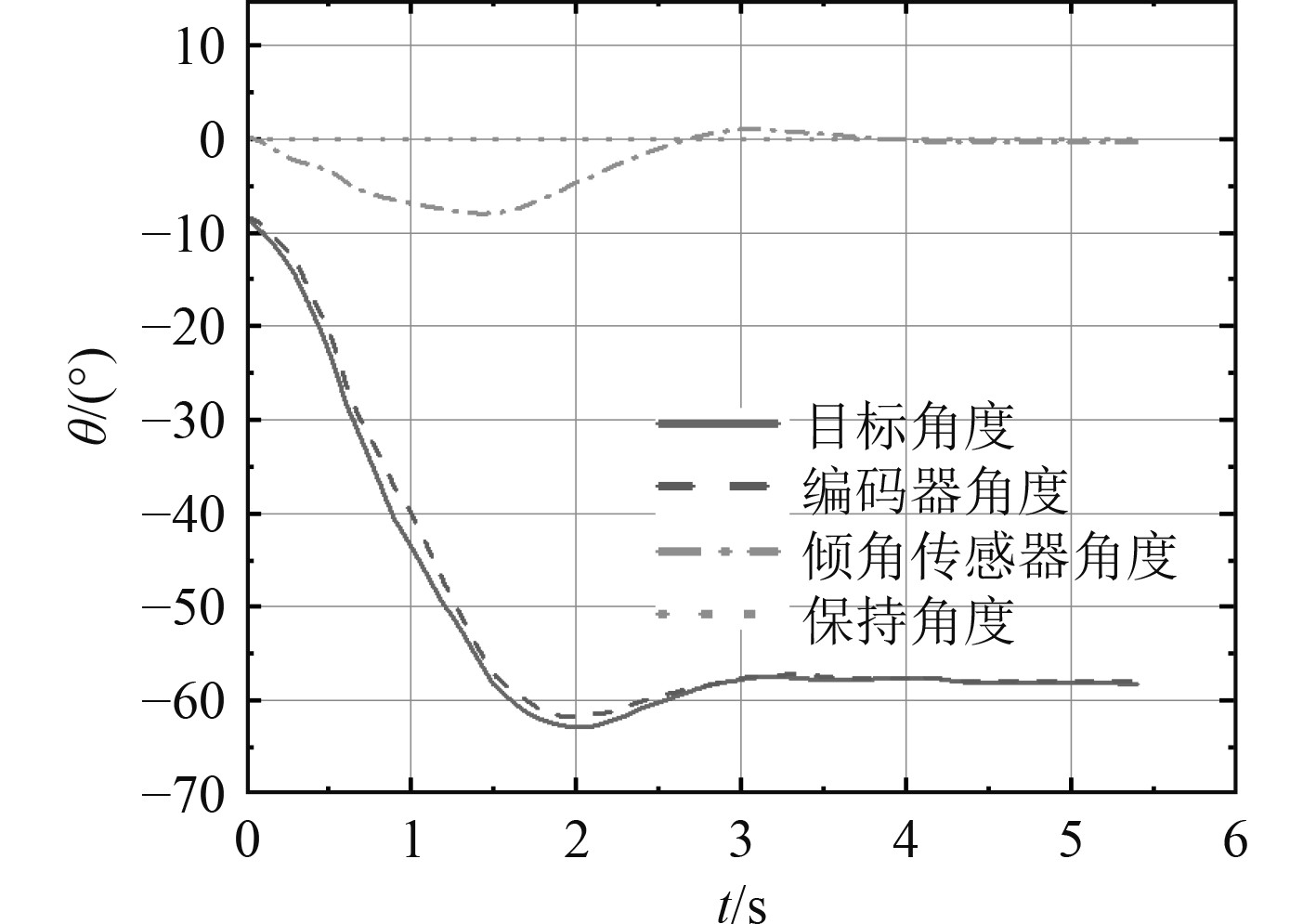图 6 Pitch轴自适应实验1 Fig. 6 The adaptive experiment 1 of Pitch

2）云台的Pitch轴初始位置位于−48.4°，上位机输入保持角度为0°后，使得D5061从50°转至−50°，得自适应角度曲线图7所示。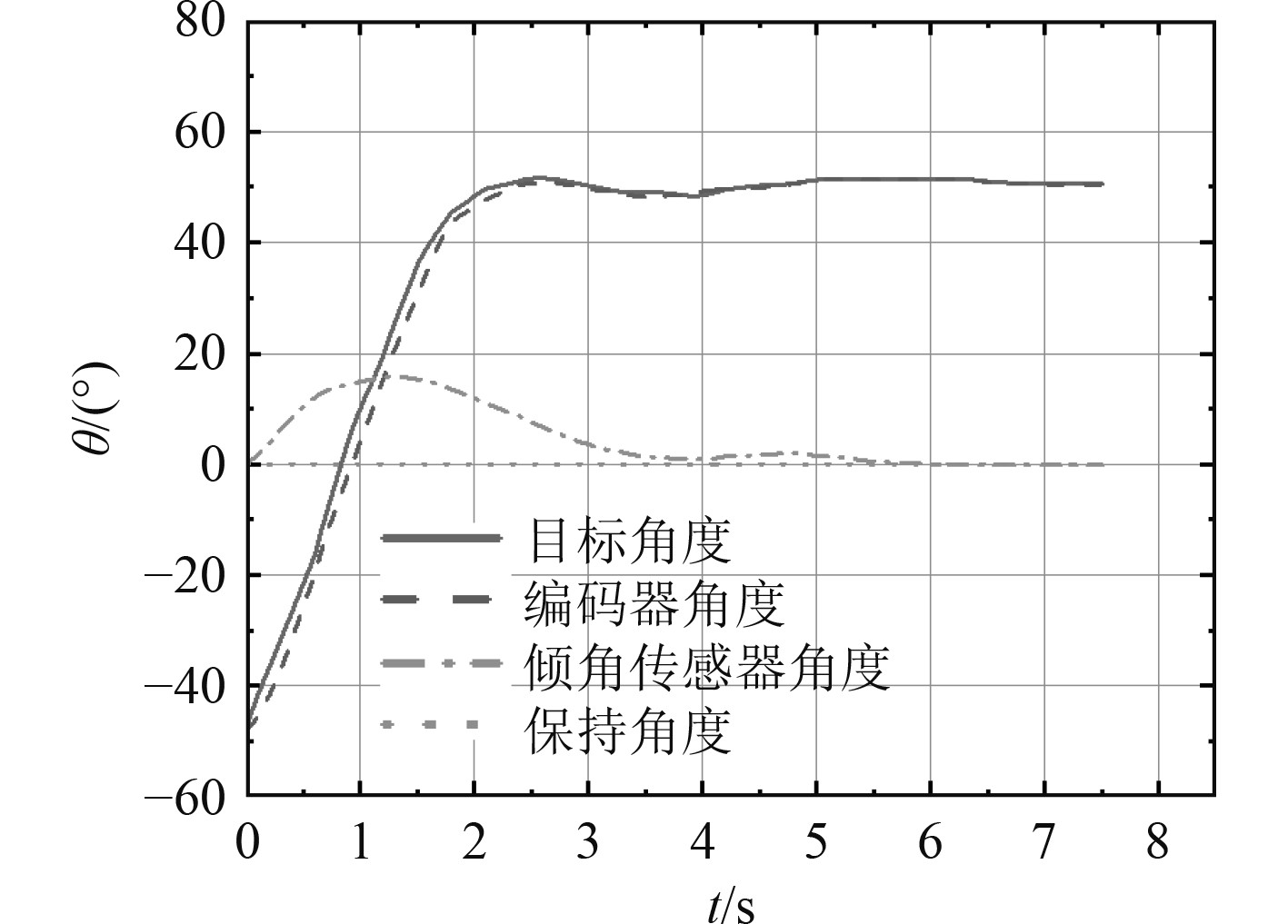图 7 Pitch轴自适应实验2 Fig. 7 The adaptive experiment 2 of Pitch

1）D5061在向某一方向转动时，云台Pitch轴往反方向转动来补偿偏移量。在此过程中，初始时D5061速度要高于云台补偿速度，倾角传感器的角度偏移量增加；在两速度相等时，倾角传感器的超调量达到最大，之后逐渐减小达到稳定。云台自调节过程中，出现微小超调后趋于稳定。系统调节时间与摆动幅度有关，但均能快速到达保持角度范围内。

2）目标角度和编码器角度曲线基本一致，在倾角传感器角度超调量较大时，曲线斜率较陡速度较大，在倾角传感器角度接近保持角度时，目标角度曲线斜率变缓直至为0，曲线变化趋势符合阶梯速度PID自适应控制方法的控制思想。

3）D5061转动50°情况下，Pitch轴稳定时间为3.5 s左右，倾角传感器最大超调量为7.9°；D5061转动100°情况下，Pitch轴在3.8 s时达到保持角度，之后出现微小超调后稳定，倾角传感器最大超调量为15.8°，系统稳定时间为5.5 s。

4）通过转动D5061模拟船左右晃动，查看Pitch轴的稳定性能。经验证，在5级海况下，系统通过自身调节稳定时间小于海浪周期，解决了声呐的姿态稳定问题，提高成像质量。

4 结　语

  宫世杰, 郭乔进, 梁中岩, 等. 一种基于飞行元数据的无人机云台控制方法[J]. 科学技术创新, 2020, 4(34): 50-51. GONG Shi-jie, GUO Qiao-jin, LIANG Zzhong-yan, et al. A control method of unmanned aerial ehicle based on flight metadata[J]. Science and Technology Innovation, 2020, 4(34): 50-51. DOI:10.3969/j.issn.1673-1328.2020.34.023  魏雅君, 高凤强, 陈冠峰, 等. 云台稳定性分析及结构优化[J]. 机械设计与研究, 2018, 34(5): 57-60.  陈杏源. 融合Camshift和粒子滤波算法的云台目标跟踪系统设计[D]. 广州: 华南理工大学, 2016.  王小平. X舵AUV控制分配优化与容错控制方法研究[D]. 哈尔滨: 哈尔滨工程大学, 2020.  陈宣成, 郭威, 周悦, 等. 全海深载人深潜器水下云台控制系统的研究与设计[J]. 制造业自动化, 2019, 41(12): 18-21+26. DOI:10.3969/j.issn.1009-0134.2019.12.005  石领先, 焦园圆. 多旋翼无人机增稳云台的结构设计[J]. 滨州学院学报, 2020, 36(6): 11-15.  林峰, 马翰廷. 机载三轴云台抗干扰方法分析[J]. 现代农机, 2020, 4(5): 30-31. DOI:10.3969/j.issn.1674-5604.2020.05.023  赵炯, 谢正东, 周奇才, 等. 二自由度云台控制系统设计及控制方法的研究[J]. 机械工程与自动化, 2020, 4(6): 21-24. ZHAO Jiong, XIE Zheng-dong, ZHOU Qi-cai, et al. Design of control system and research on control method of ptz with two degrees of freedom[J]. Mechanical Engineering and Automation, 2020, 4(6): 21-24. DOI:10.3969/j.issn.1672-6413.2020.06.008  闫磊, 王萌, 朱烨繁. 双目四自由度视觉云台模糊自适应PID目标跟踪控制[J]. 控制工程, 2021, 28(5): 1-8. YAN Lei, WANG Meng, ZHU Ye-fan. Binocular 4-DOF vision PTZ fuzzy adaptive PID target tracking control[J]. Control Engineering of China, 2021, 28(5): 1-8.  HESHMATI-ALAMDARI S, NIKOU A, KYRIAKOPOULOS K J, et al. A robust force control approach for underwater vehicle manipulator systems[J]. International Federation of Automatic Control, 2021, 50(1): 11197-11202.  BORLAUG I, SVERDRUP-THYGESON J, PETTERSEN K Y, et al. Combined kinematic and dynamic controlof an underwater swimming manipulator[J]. International Federation of Automatic Control, 2019, 52(21): 8-13.  SVERDRUP-THYGESON J, KELASIDI E, PETTERSEN K Y, et al. A control framework for biologically inspiredunderwater swimming manipulators equipped with thrusters[J]. International Federation of Automatic Control, 2016, 49(23): 89-96.  SATJA S, JOSEPH C, EDIN O, et al. Underwater manipulators: a review[J]. Ocean Engineering, 2018, 163: 431-450. DOI:10.1016/j.oceaneng.2018.06.018  刘喜藏, 林皓, 张玲. 航母在不同海况下的运动仿真研究[C]// 2020中国航空工业技术装备工程协会年会论文集, 《测控技术》杂志社, 2020: 324–327.